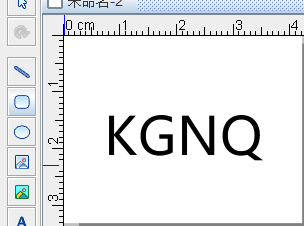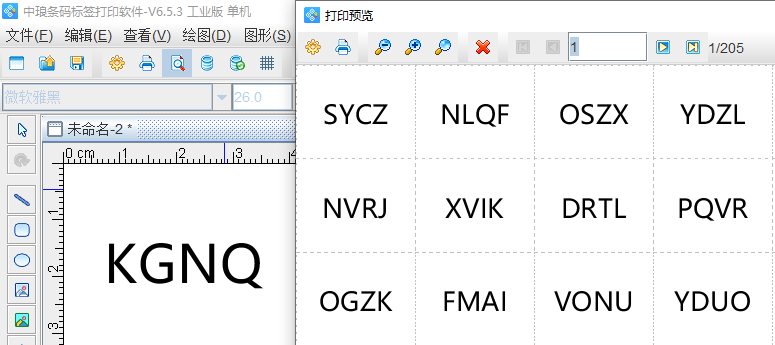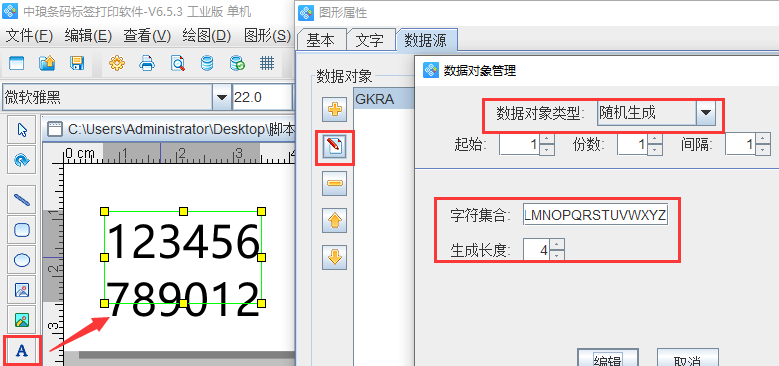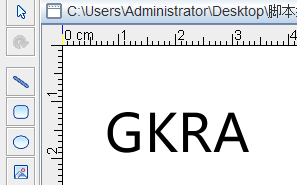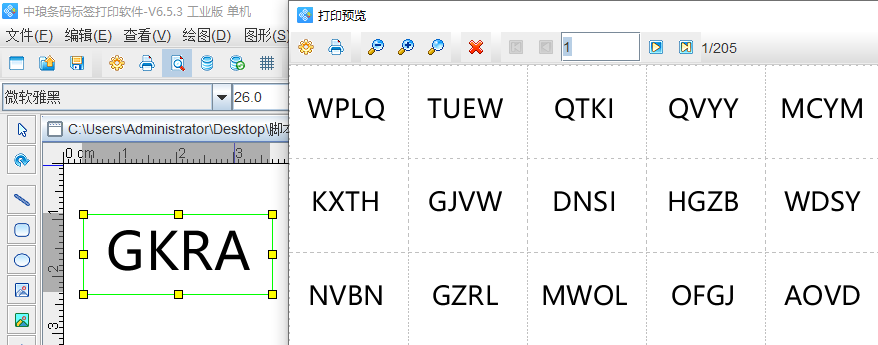# 如何用脚本编程随机产生字母点击图片查看原图

 需求数量： 价格要求： 包装要求： 所在地： 河南郑州市 有效期至： 长期有效 最后更新： 2023-09-13 11:32近来有客户说，在条码打印软件网站介绍了如何用脚本编程随机产生数字，他们现在碰到了一个问题是，如何用脚本编程随机产生字母。其实用随机生成也可以随机生成字母，只需要修改字符集合及生成长度即可，但是客户不想用随机生成，要求用脚本编程，咨询该如何设置，方法如下：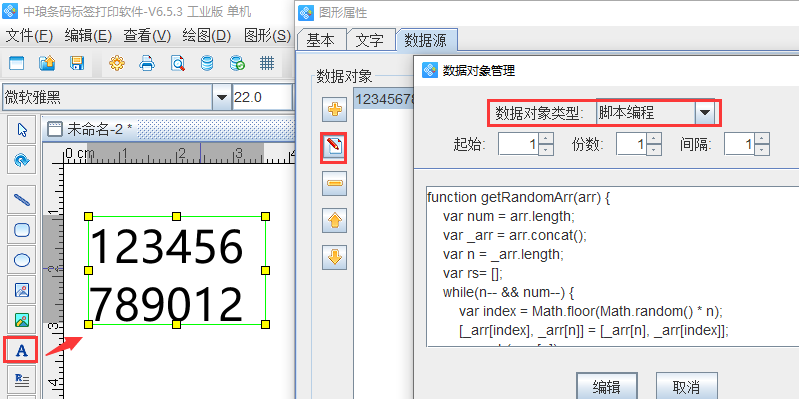function getRandomArr(arr) {
var num = arr.length;
var _arr = arr.concat();
var n = _arr.length;
var rs= [];
while(n-- && num--) {
var index = Math.floor(Math.random() * n);
[_arr[index], _arr[n]] = [_arr[n], _arr[index]];
rs.push(_arr[n]);
}
return rs;
}
var letters = "ABCDEFGHIJKLMNOPQRSTUVWXYZ".split("");
var result=[];
ran = Math.floor(Math.random() * letters.length);
result.push(letters.splice(ran, 1));
ran = Math.floor(Math.random() * letters.length);
result.push(letters.splice(ran, 1));
ran = Math.floor(Math.random() * letters.length);
result.push(letters.splice(ran, 1));
ran = Math.floor(Math.random() * letters.length);
result.push(letters.splice(ran, 1));
result = getRandomArr(result);
strReturn=result.join("");